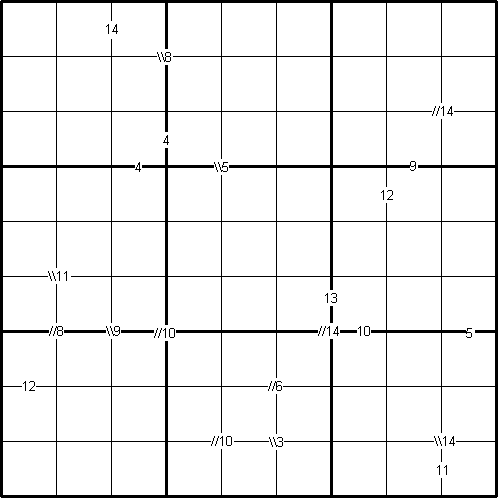# Integrated Sums Sudoku

##### Age 11 to 16Challenge Level

by Henry Kwok### The Rules of "Integrated Sums Sudoku"

Like the standard Sudoku, this Sudoku variant consists of a grid of nine rows and nine columns subdivided into nine 3$\times$3 subgrids. Like the standard Sudoku, it has two basic rules:
1. Each column, each row, and each box (3$\times$3 subgrid) must have the numbers 1 to 9.
2. No column, row or box can have two squares with the same number.
The puzzle can be solved with the help of small clue-numbers which are either placed on the border lines between selected pairs of neighbouring squares of the grid or placed after slash marks on the intersections between two diagonally adjacent squares.

Each small clue-number is the sum of two digits in the two squares that are horizontally or vertically or diagonally adjacent. The position of each pair of diagonally adjacent squares is indicated by either two forward slash marks // or two backward slash marks \\.

For example, the //14 on the intersection between the diagonally adjacent squares (2, 9) and (3, 8) means that possible pairs of numbers in the squares are: 5 and 9, 9 and 5; 6 and 8, or 8 and 6 respectively.

The \\5 on intersection between the diagonally adjacent squares (3, 4) and (4, 5) means that possible pairs of numbers in the squares are: 1 and 4, 4 and 1; 2 and 3, or 3 and 2 respectively.

Finally, the clue-number 12 on the border line betweeen the squares (4, 7) and (4, 8) means that possible pairs of numbers for these squares can be from the following combinations: 3 and 9, 9 and 3; 4 and 8, 8 and 4; 5 and 7, or 7and 5.